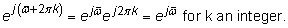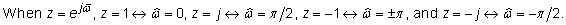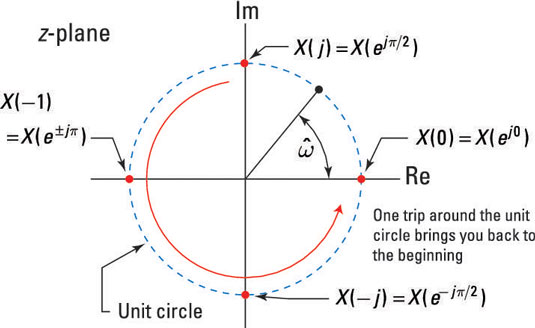##### Signals and Systems For Dummies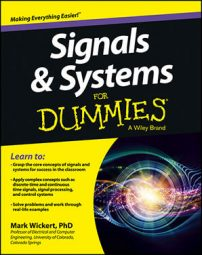The z-transform (ZT) is a generalization of the discrete-time Fourier transform (DTFT) for discrete-time signals, but the ZT applies to a broader class of signals than the DTFT. The two-sided or bilateral z-transform (ZT) of sequence x[n] is defined as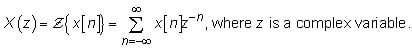The ZT operator transforms the sequence x[n] to X(z), a function of the continuous complex variable z. The relationship between a sequence and its transform is denoted as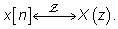You can establish the connection between the discrete-time Fourier transform (DTFT) and the ZT by first writing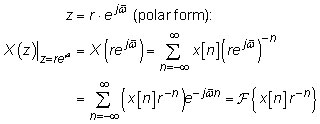The special case of r = 1 evaluates X(z) over the unit circle —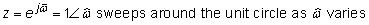and is represented as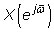the DTFT of x[n]. This result holds as long as the DTFT is absolutely summable (read: impulse functions not allowed).

The view that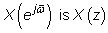sampled around the unit circle in the z-plane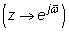shows that the DTFT has period 2π because